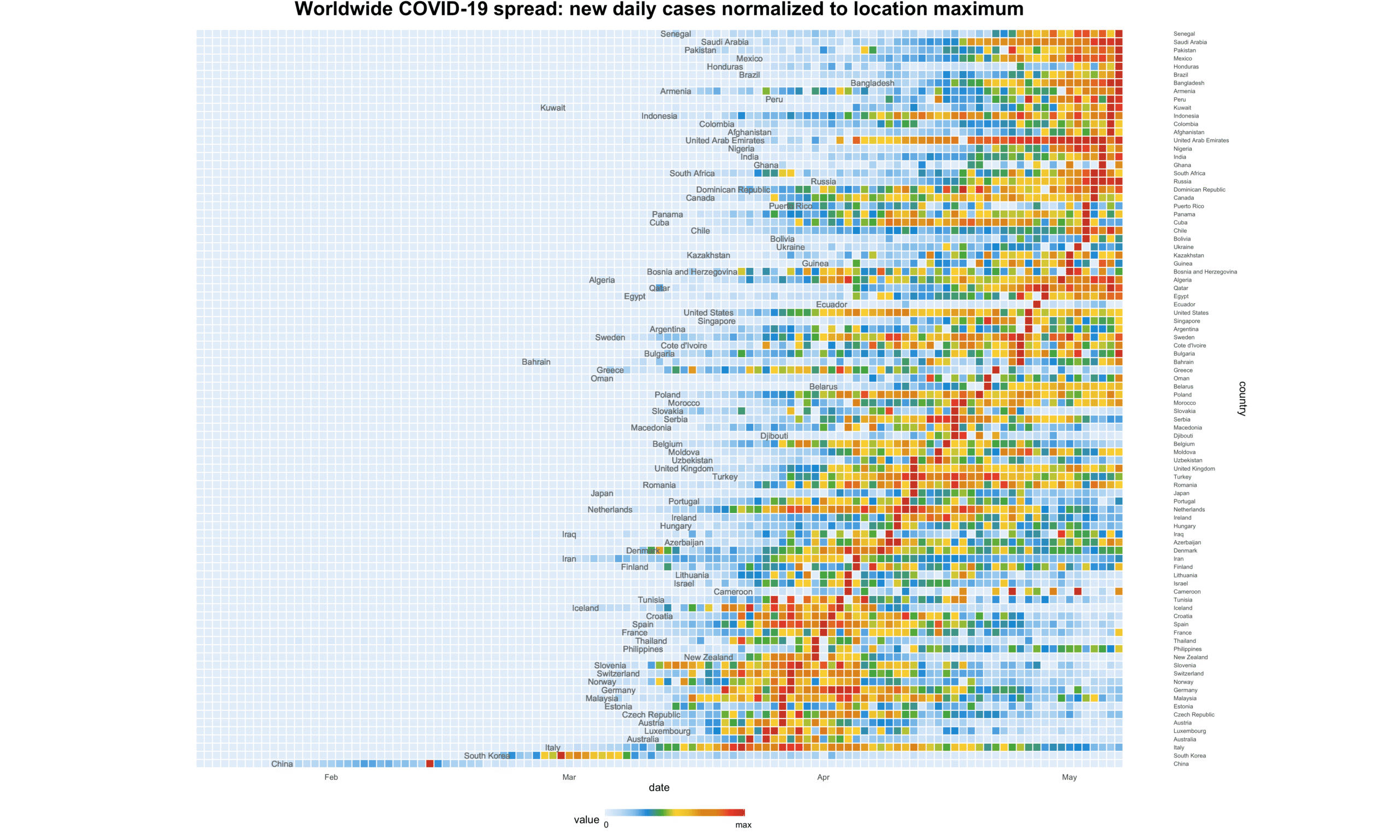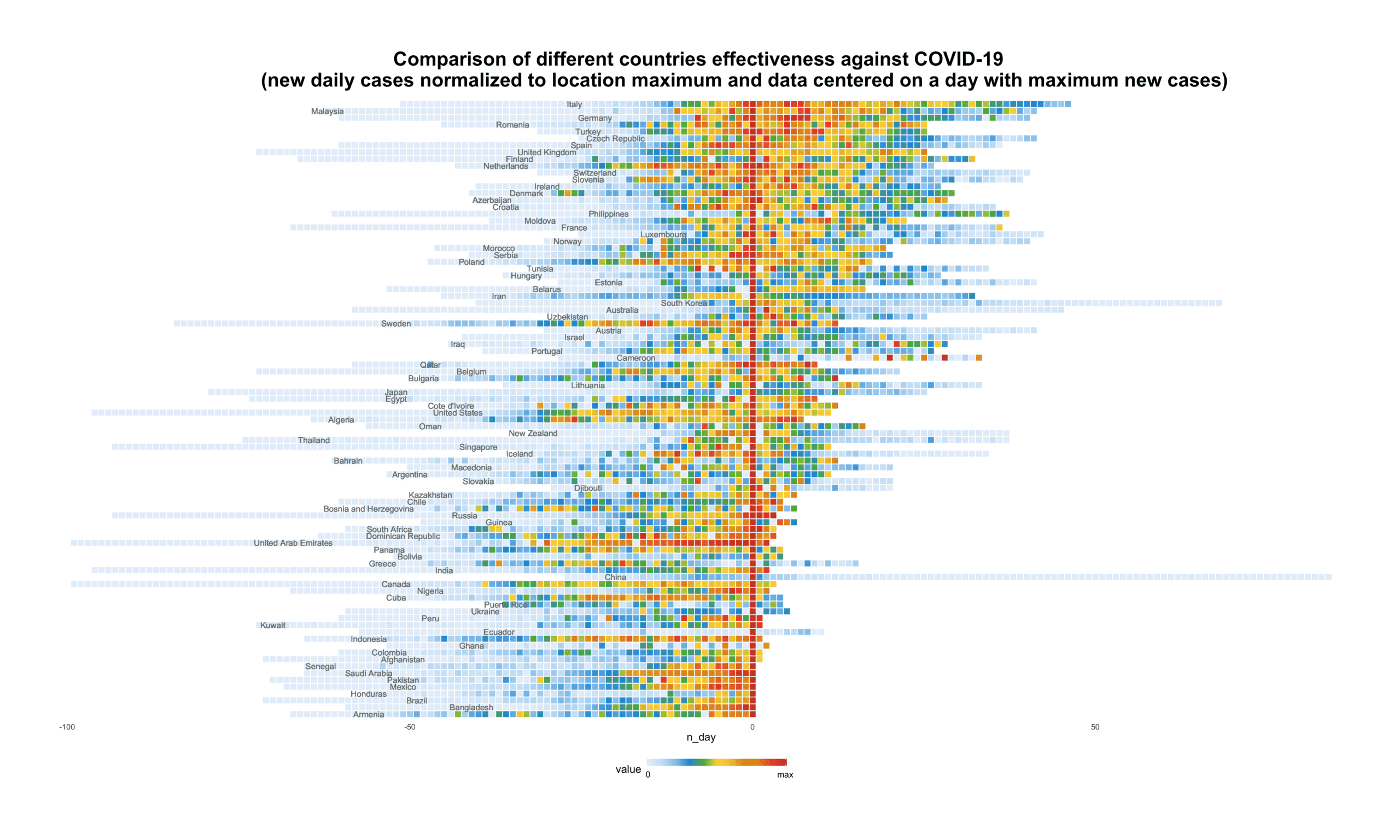Back to Home

Worldwide COVID-19 spread visualization with R

COVID-19 or Coronavirus pandemic is having an unpredictable and huge impact on our lives, so I wanted to see the speed with which it spreads across countries. The following is how I’ve seen it:

This animated visualization focuses on the chronology of the virus spreading from China to the rest of the World. In order to strengthen the visual effect, I placed the top 90 countries in two semi diagonals, based on the date when each country reached the daily cases peak (dark red box).

For a more detailed analysis, I’ve created two stationary charts. The first is the same as the animated one but countries are ordered from bottom to top.The second one focuses on peaks and shows how long and intensive the previous and following stages were. It provides an opportunity to compare each countries’ effectiveness.All values of new cases for each country were normalized via min/max normalization and ranged from 0 to 1. You can use the following R code with comments to play with the public dataset:

click to expand R code
```library(tidyverse)
library(reshape2)
library(purrrlyr)

# normalization function
fun_normalize <- function(x) {
return ((x - min(x)) / (max(x) - min(x)))
}

# preprocess data
df_prep <- df %>%
filter(location != 'World') %>%

group_by(location) %>%
# remove earlier dates
filter(date > as.Date('2020-01-15', format = '%Y-%m-%d')) %>%
# remove coutries with less than 1000 total cases
filter(max(total_cases) > 1000) %>%
# replace negative values with the mean
mutate(new_cases = ifelse(new_cases < 0,
round((lag(new_cases, default = 0) + lead(new_cases, default = 0)) / 2),
new_cases)) %>%
ungroup() %>%
select(location, date, new_cases) %>%
# prepare data for normalization
dcast(., date ~ location, value.var = 'new_cases') %>%
# replace NAs with 0
dmap_at(c(2:ncol(.)), function(x) ifelse(is.na(x), 0, x)) %>%
# normalization
dmap_at(c(2:ncol(.)), function(x) fun_normalize(x)) %>%
melt(., id.vars = c('date'), variable.name = 'country') %>%
mutate(value = round(value, 6))

# define countries order for plots
country_ord_1 <- df_prep %>%
group_by(country) %>%
filter(value == 1) %>%
ungroup() %>%
arrange(date, country) %>%
distinct(country) %>%
mutate(is_odd = ifelse((row_number() - 1) %% 2 == 0, TRUE, FALSE))

country_ord_anim <- bind_rows(country_ord_1 %>%
filter(is_odd == TRUE) %>%
arrange(desc(row_number())),
country_ord_1 %>%
filter(is_odd == FALSE))

# data for animated plot
df_plot_anim <- df_prep %>%
mutate(country = factor(country, levels = c(as.character(country_ord_anim\$country)))) %>%
group_by(country) %>%
mutate(first_date = min(date[value >= 0.03])) %>%
mutate(cust_label = ifelse(date >= first_date, as.character(country), '')) %>%
ungroup()

# color palette
cols <- c('#e7f0fa','#c9e2f6', '#95cbee', '#0099dc', '#4ab04a', '#ffd73e', '#eec73a', '#e29421', '#e29421', '#f05336', '#ce472e')

# Animated Heatmap plot
p <- ggplot(df_plot_anim, aes(y = country, x = date, fill = value)) +
theme_minimal() +
geom_tile(color = 'white', width = .9, height = .9) +
scale_fill_gradientn(colours = cols, limits = c(0, 1),
breaks = c(0, 1),
labels = c('0', 'max'),
guide = guide_colourbar(ticks = T, nbin = 50, barheight = .5, label = T, barwidth = 10)) +

geom_text(aes(x = first_date, label = cust_label), size = 3, color = '#797D7F') +
scale_y_discrete(position = 'right') +
coord_equal() +

theme(legend.position = 'bottom',
legend.direction = 'horizontal',
plot.title = element_text(size = 20, face = 'bold', vjust = 2, hjust = 0.5),
axis.text.x = element_text(size = 8, hjust = .5, vjust = .5, face = 'plain'),
axis.text.y = element_blank(),
axis.title.y = element_blank(),
panel.grid.major = element_blank(),
panel.grid.minor = element_blank()
) +
ggtitle('Worldwide COVID-19 spread: new daily cases normalized to location maximum')

# animated chart
library(gganimate)
library(gifski)

anim <- p +
transition_components(date) +
ggtitle('Worldwide COVID-19 spread: new daily cases normalized to location maximum',
subtitle = 'Date {frame_time}') +

animate(anim,
nframes = as.numeric(difftime(max(df_plot_anim\$date), min(df_plot_anim\$date), units = 'days')) + 1,
duration = 12,
fps = 12,
width = 1000,
height = 840,
start_pause = 5,
end_pause = 25,
renderer = gifski_renderer())
anim_save('covid-19.gif')

# Heatmap plot 1
df_plot_1 <- df_prep %>%
mutate(country = factor(country, levels = c(as.character(country_ord_1\$country)))) %>%
group_by(country) %>%
mutate(first_date = min(date[value >= 0.03])) %>%
ungroup()

ggplot(df_plot_1, aes(y = country, x = date, fill = value)) +
theme_minimal() +
geom_tile(color = 'white', width = .9, height = .9) +
scale_fill_gradientn(colours = cols, limits = c(0, 1),
breaks = c(0, 1),
labels = c('0', 'max'),
guide = guide_colourbar(ticks = T, nbin = 50, barheight = .5, label = T, barwidth = 10)) +

geom_text(aes(x = first_date, label = country), size = 3, color = '#797D7F') +
scale_y_discrete(position = 'right') +
coord_equal() +

theme(legend.position = 'bottom',
legend.direction = 'horizontal',
plot.title = element_text(size = 20, face = 'bold', vjust = 2, hjust = 0.5),
axis.text.x = element_text(size = 8, hjust = .5, vjust = .5, face = 'plain'),
axis.text.y = element_text(size = 6, hjust = .5, vjust = .5, face = 'plain'),
panel.grid.major = element_blank(),
panel.grid.minor = element_blank()
) +
ggtitle('Worldwide COVID-19 spread: new daily cases normalized to location maximum')

# Heatmap plot 2
df_plot_2 <- df_prep %>%
group_by(country) %>%
filter(date >= min(date[value > 0])) %>%
arrange(date, .by_group = TRUE) %>%
mutate(centr_day = min(row_number()[value == 1]),
n_day = row_number() - centr_day) %>%
ungroup()

country_ord_2 <- df_plot_2 %>%
group_by(country) %>%
filter(date >= min(date[value == 1])) %>%
summarise(value = sum(value)) %>%
ungroup() %>%
arrange(value, country) %>%
distinct(country)

df_plot_2 <- df_plot_2 %>%
mutate(country = factor(country, levels = c(as.character(country_ord_2\$country)))) %>%
group_by(country) %>%
mutate(first_date = min(n_day[value >= 0.01])) %>%
ungroup()

# Heatmap plot 2
ggplot(df_plot_2, aes(y = country, x = n_day, fill = value)) +
theme_minimal() +
geom_tile(color = 'white', width = .9, height = .9) +
scale_fill_gradientn(colours = cols, limits = c(0, 1),
breaks = c(0, 1),
labels = c('0', 'max'),
guide = guide_colourbar(ticks = T, nbin = 50, barheight = .5, label = T, barwidth = 10)) +

geom_text(aes(x = first_date, label = country), size = 3, color = '#797D7F') +
coord_equal() +

theme(legend.position = 'bottom',
legend.direction = 'horizontal',
plot.title = element_text(size = 20, face = 'bold', vjust = 2, hjust = 0.5),
axis.text.x = element_text(size = 8, hjust = .5, vjust = .5, face = 'plain'),
#axis.text.y = element_text(size = 6, hjust = .5, vjust = .5, face = 'plain'),
axis.text.y = element_blank(),
axis.title.y = element_blank(),
panel.grid.major = element_blank(),
panel.grid.minor = element_blank()
) +
ggtitle('Comparison of different countries effectiveness against COVID-19
(new daily cases normalized to location maximum and data centered on a day with maximum new cases)')

```

Get new post notification